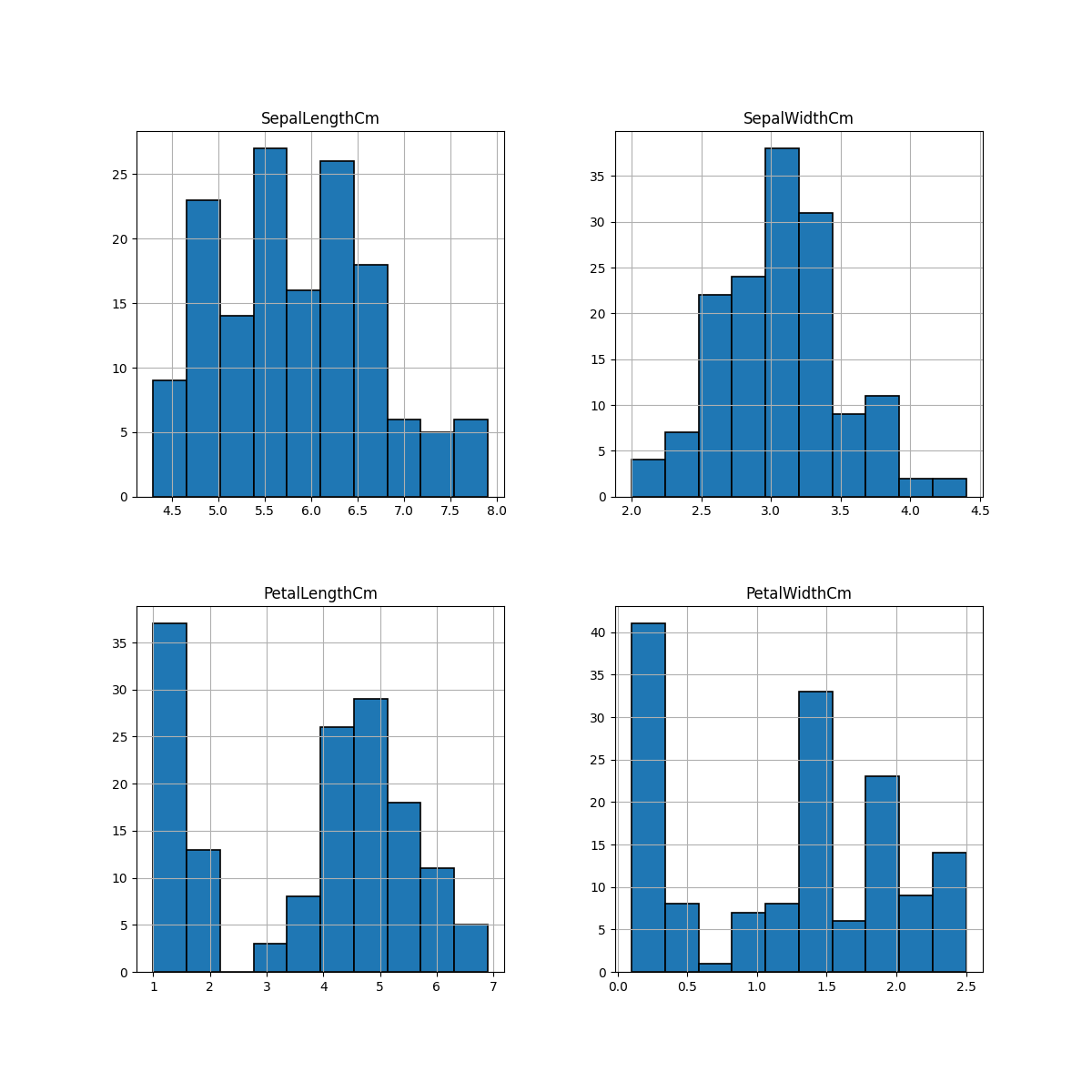﻿ Python Machine learning Scikit-learn: Create a graph to see how the length and width of SepalLength, SepalWidth, PetalLength, PetalWidth are distributed - w3resource# Python Scikit-learn: Create a graph to see how the length and width of SepalLength, SepalWidth, PetalLength, PetalWidth are distributed

## Python Machine learning Iris Visualization: Exercise-6 with Solution

Write a Python program to create a graph to see how the length and width of SepalLength, SepalWidth, PetalLength, PetalWidth are distributed.

Sample Solution:

Python Code:

``````import pandas as pd
import matplotlib.pyplot as plt
iris = pd.read_csv("iris.csv")
# Drop id column
new_data = iris.drop('Id',axis=1)
new_data.hist(edgecolor='black', linewidth=1.2)
fig=plt.gcf()
fig.set_size_inches(12,12)
plt.show()
```
```

Output:

``````

Python Code Editor:

Have another way to solve this solution? Contribute your code (and comments) through Disqus.

What is the difficulty level of this exercise?

﻿